# String searching

The string searching or string matching problem is that of locating one string, known as a needle or a pattern, as a substring of another, longer string, known as a haystack or the text. (These terms are derived from the idiom finding a needle in a haystack.) It is precisely the function implemented by the "Find" feature in a text editor or web browser. Because of the information explosion and the fact that much of the information in the world exists in the form of text, efficient information retrieval by means of string searching is instrumental in a wide variety of applications. Consequently, this problem is one of the most extensively studied in computer science. The more general problem of determining whether a text contains a substring of a particular form, such as a regular expression, is known as pattern matching.

## Discussion

In theory, the string searching problem could be formulated as a decision problem, the problem of whether or not the needle is a substring of the haystack. However, this variation is often not used in practice, since we almost always want to know where the needle occurs in the haystack, and none of the important string searching algorithms make it difficult to determine this once they have established that the substring exists. (For example, the string mat appears twice in mathematics, once starting from the first character, and once starting from the sixth character.) On the other hand, sometimes we will only care about the first or the last position at which the needle occurs in the haystack, and other times we will want to find all positions at which it matches.

If a string searching algorithm is to be used on only one needle-haystack pair, its efficiency is determined by two factors: the length of the needle,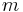$m$, and the length of the haystack,$n$. Several algorithms achieve the asymptotically optimal$\Theta(m+n+p)$ worst-case runtime here (where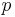$p$ is the number of matches found for the needle in the haystack), which is linear time.

We will sometimes want to search for one pattern in multiple texts; the "Search" feature of your operating system, for example, will accept one search string and attempt to locate it in multiple files. Using any single-pattern, single-text linear-time string searching algorithm for this case will also give an asymptotically optimal linear-time algorithm, as it will use$O(m + n_1 + m + n_2 + ...)$ time, which is the same as$O(n_1 + n_2 + ...)$ since the needle is never longer than the haystack (and hence the sum of the needle and haystack lengths is never more than twice the haystack length). That is, preprocessing the needle will not give a multiple-text string searching algorithm that is asymptotically faster than running an asymptotically optimal single-pattern, single-text algorithm once on each text.

The situation is very different when we want to search for multiple patterns in one text. As an example, a biologist may frequently wish to locate genes in the human genome. This 3.3 billion-character text produced through the efforts of the Human Genome Project does not change, but the there are undoubtedly a great number of different genes (patterns) that biologists may wish to locate in it. Here, if we run a single-pattern, single-text algorithm for each pattern, we have to consider the entire text each time. When the text is much longer than the patterns, the behavior of this strategy is much worse than linear time. It is possible to preprocess the text so that it will not be necessary to iterate through the entire text each time a new pattern is searched for within it. This is known as indexing.

## Naive algorithm

A straightforward string search algorithm looks as follows. Let the characters of the needle be denoted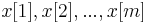$x, x, ..., x[m]$ and those of the haystack$y, y, ..., y[n]$:

```for i ∈ [0..n-m]
match ← true
for j ∈ [1..m]
if x[j] = y[i+j]
match ← false
break
if match
{needle found starting at position i+1 of haystack}
```

That is, every possible position in the haystack is considered a potential match and tested one character at a time to determine whether it is an actual match.

Although this is a naive algorithm, in practice it is likely to run quite quickly when the needle and haystack are essentially random. This is because the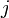$j$th character of the potential match will be examined if and only if the previous$j-1$ characters matched, which is expected to occur with probability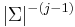$|\Sigma|^{-(j-1)}$. Thus, in the infinite limit, the expected number of characters examined for each partial match is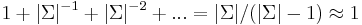$1 + |\Sigma|^{-1} + |\Sigma|^{-2} + ... = |\Sigma|/(|\Sigma|-1) \approx 1$ when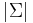$|\Sigma|$, the alphabet size, is large. This is equivalent to saying that mismatches will usually be found quite early, giving a linear runtime.

Unfortunately, this analysis is far too optimistic, because searching for a completely random needle in a completely random haystack is not very useful. In practice, when searching, we will often already know that the needle is somewhere within the haystack. If using the "Search and Replace" feature of a text editor, we often know the needle occurs several times within the haystack. Under these circumstances, the naive algorithm is likely to perform much worse. A worst case occurs when, for example, the needle is AAAAAAA and the haystack is AAAAAAAAAAAA. Here, seven positions of the haystack have to be checked, and in each case all seven characters of the substring beginning there have to be checked to verify the potential match. In general, the naive algorithm has running time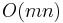$O(mn)$, which is far too slow for many real-world applications involving vast quantities of text.

## Knuth–Morris–Pratt algorithm

To see how the naive algorithm can be improved, consider two examples. The first is the worst-case example of searching for a string of seven A's in a string of thirteen A's. The naive algorithm will first check the substring of the haystack located from positions 1 to 7, inclusive, and find a match. Then it will check the substring in positions 2 to 8, inclusive, and so on. But no human would search this way, because in this case, the first match tells us that the characters from positions 2 to 7 are all A's, which means that instead of re-checking all the characters from positions 2 to 8 we only need to check the eighth character. As long as we keep finding A's, each of them signals a new match, and we never have to look back.

Furthermore, when we encounter a different character in the haystack, such as B, as in the string AAAAAABAAAAAAA, this gives us useful information as well. In particular, since the character B occurs at position 7 in the haystack, and the needle contains no Bs at all, after a failed match at positions 1 to 7, we can immediately rule out the possibilities of finding matches at positions 2 to 8, 3 to 9, 4 to 10, 5 to 11, 6 to 12, and 7 to 13, and continue our search starting from character 8.

Another example: suppose we are searching for the string tartan in the string tartaric_acid. We make the observation that, in the needle, the ta starting at the fourth position is identical to the ta starting at the first position. Now, when we perform the search, we start by testing the substring tartar (i.e., at position 1 of the haystack); we see the the first five characters match but that we then have a mismatch with tartan and tartaric_acid. At this point, we first notice that we already have some partial match information. In particular, we can rule out the second and third positions of the haystack, and when we start trying to match the fourth position (the substring taric_), there is no need to start from the t, because we know that this ta already matches the tartar. We know this because when trying to match from the first position of tartaric_acid we found a match between tartan and tartaric_acid, i.e., tartan matches tartaric_acid, and hence tartan matches tartaric acid (since we have already established that the substring ta occurs at both the first and the fourth positions of tartan). In this way we avoid examining the fourth and fifth characters of the haystack more than once.

It may seem that a bit of human intelligence has been used in these examples, but it turns out that this "intelligence" is of a form that can be easily and exactly implemented on a computer. The needle is preprocessed in such a way that it becomes possible to eliminate potential positions after a failed match and to avoid re-checking characters on successful matches, and after a character from the haystack is examined, previous characters never need to be examined again. Furthermore, we do not have to store large substring match tables; instead, we can do it with linear time and linear space, giving an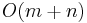$O(m+n)$ algorithm overall. This is known as the Knuth–Morris–Pratt algorithm (KMP). This algorithm, however, is not suitable for searching for several needles in one haystack.

## Rabin–Karp algorithm

Another approach is based on hashing. In particular, we first hash the needle and then hash each substring of the haystack of length$m$ (using the same hash function). By comparing the hash of each of these substrings of the haystack with the hash of the needle, we can rule out many positions in the haystack (i.e., when the hashes do not match).

The problem with this approach as presented is that a good hashing algorithm always examines all of the data, i.e., the entire substring of the potential match. If this is the case, then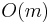$O(m)$ time will be spent hashing each potential match, giving a runtime no better than that of the naive algorithm.

Instead, we use a rolling hash. This is a hash function with the useful property that if we already know the hash of the substring in positions$i+1, i+2, ..., i+m$ of the haystack, then we can compute the hash of the substring$i+2, i+3, ..., i+m+1$ in constant time. (After all, only two characters actually change — one added and one removed — and the rest just slide over). The Rabin–Karp algorithm can be formulated either as a Monte Carlo algorithm (in which we assume that a match in hashes is an actual match, without bothering to verify the potential match, giving a linear runtime and a small probability of failure) or a Las Vegas algorithm (in which we verify each match in hashes as an actual match using the naive method, so that in the worst case in which every position is a match, we still get the naive$O(mn)$ runtime, but we will always be correct) but not as a provably guaranteed correct and efficient algorithm. For this reason, the Rabin–Karp algorithm is useful in programming contests, but rarely deployed in real-world applications. The Rabin–Karp algorithm does have the ability to efficiently search for multiple needles in one haystack by simply hashing each needle separately then checking whether the rolling hash of the haystack at each step matches any of the needle hashes. By storing the needle hashes in a hash table, we obtain linear time.

## Aho–Corasick algorithm

The Aho–Corasick algorithm is more complex than KMP and Rabin–Karp, but it has the desirable property of exactly solving the multiple-pattern string search problem in linear time (unlike KMP, which is slow for multiple-pattern search, and Rabin–Karp, which has a small probability of either being slow or being incorrect). It builds a finite automaton which at its core is a trie of entire set of patterns. The text is processed one character at a time, without looking back (as in KMP). Each node in the trie remembers which, if any, of the patterns are a suffix of the string represented by this node, so that once we have processed a character and passed through this node, we will know whether any substring ending at this character matches any of the patterns. If the current character in the text matches a branch from our current position in the trie, we proceed down that branch, as all the patterns in that branch are still potential matches. In case of a mismatch, we proceed to the longest possible suffix of the string to which the current position in the trie corresponds, which is made possible by augmenting the trie with suffix links.

The Aho–Corasick algorithm is suitable when the set of patterns to be searched for is known in advance. For example, we might want a search engine to return all pages that contain all of the given search terms. This can be done by constructing the Aho–Corasick automaton of the search terms given and then executing a single search pass on each page. However, this might not always be the case; the example previously given was that of a webserver that locates genes within the human genome. Unless different biologists agree to batch together their queries, the Aho–Corasick algorithm will perform no better than KMP in this application.

## Suffix data structures

The final solution to multiple-pattern searching is given by data structures that index the suffixes of a string, namely, the suffix tree and the suffix array. The suffix tree is a compressed trie of all the suffixes of a given string. We can search for a substring simply by walking down starting from the root; if a corresponding branch ultimately does not exist then the needle is not found, and if it does, then all matches are to be found in the subtree rooted at the node we end up at. The suffix tree can be constructed in linear time, although the algorithm to do so is very complex; but constructing a suffix tree of the haystack allows a needle to be searched for in time proportional to its length (plus the number of matches, if we wish to return all of them). The suffix array is a sorted list of the suffixes of the string; if the needle is a prefix of any of the suffixes of the haystack then it is found at the positions at which those suffixes start, and clearly the matches will occur in a contiguous segment of the suffix array which can be determined using binary search.

## Boyer–Moore algorithm

The Boyer–Moore algorithm is, on average, the fastest algorithm for single-pattern search. In the worst case it runs in linear time, but in the average case it examines only a fraction of the characters in the haystack. It does so by preprocessing the needle and then attempting to match the needle in reverse order while still considering possible match positions in the haystack in forward order. This allows it to rule out potential matches as soon as possible. For example, recall the example of looking for the needle AAAAAAA in the haystack AAAAAABAAAAAAA. Using KMP, we first match the first six characters of the needle to the first six characters of the haystack, at which point we discover that the seventh characters do not match. This allows us to rule out six other positions at which the needle might be found, so that we can resume processing the haystack at the first position after the B. The Boyer–Moore algorithm, on the other hand, would start by comparing AAAAAAA with AAAAAABAAAAAAA, that is, by trying to match the last character of the needle. (If they match, then it would proceed to compare the second-last characters, and so on.) It would then find the mismatch immediately, and, using the information determined in the preprocessing stage, conclude the same information as KMP — that the search should resume starting at the eighth position of the haystack, at which point it would start matching from the end again, examining the fourteenth character first and then ultimately returning to the eighth character of the haystack and the first character of the needle, at which point a match is confirmed. Notice that Boyer–Moore did not even bother to examine the first six characters of the haystack. On average, then, the Boyer–Moore algorithm tends to run in time linear in the length of the needle plus sublinear in the length of the haystack.